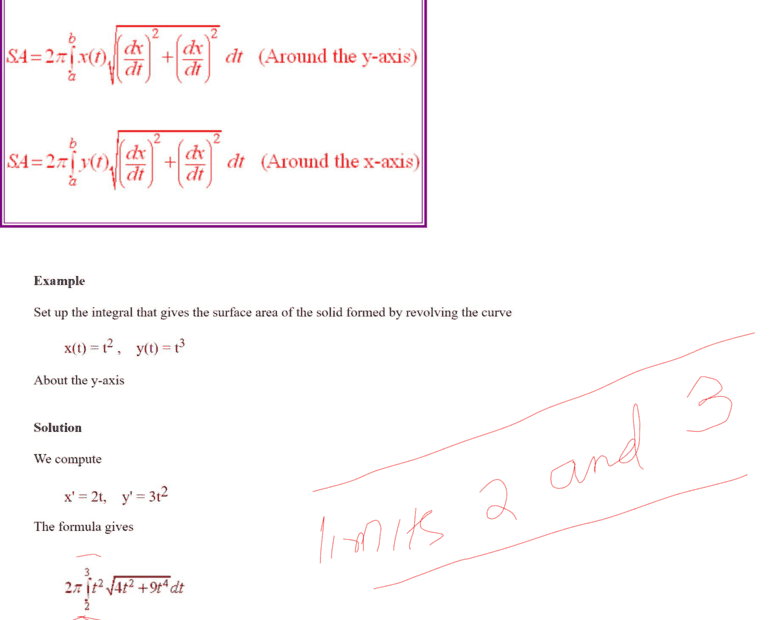# Find the surface area of the given solid

• chwala

#### chwala

Gold Member
Homework Statement
See attached
Relevant Equations
Parametric equations
My question is on how did they determine the limits of integration i.e ##2## and ##3## as highlighted? ThanksThe problem statement is incomplete.

I meant that the problem statement did not include the limits, i.e., poorly written.

Last edited:
•chwala
Frabjous said:
I meant that the problem statement did not include the limits, i.e., poorly written.
I agree. The graph of the parametric curve ##x = t^2, y = t^3## lies in Quadrants I and IV, and is unbounded. There has to be additional but unstated constraints for the limits of integration that are shown.

•chwala
@chwala : It seems in your square root, you're using ##\frac {dx}{dt} ##twice, rather than what I believe is correct, ##\frac {dx}{dt}, \frac{dy}{dt}##

WWGD said:
@chwala : It seems in your square root, you're using ##\frac {dx}{dt} ##twice, rather than what I believe is correct, ##\frac {dx}{dt}, \frac{dy}{dt}##
@WWGD This is not my working rather notes that i came across as indicated by the given internet link;

yes, there is a mistake there... it ought to be

$$Surface area (y-axis) = 2π \int_ a^b x(t)\sqrt{(x^{'})^2+(y{'})^2}$$

where

$$x^{'}=\dfrac{dx}{dt}$$

$$y^{'}=\dfrac{dy}{dt}$$

•WWGD
Since there seems to be two errors on that page, the missing information about boundaries, and the formula for surface area, perhaps you should look elsewhere for information on how to calculate surface area.

•chwala